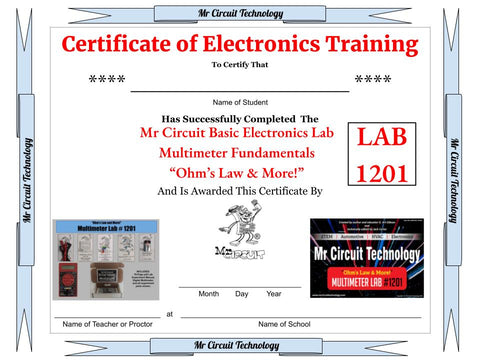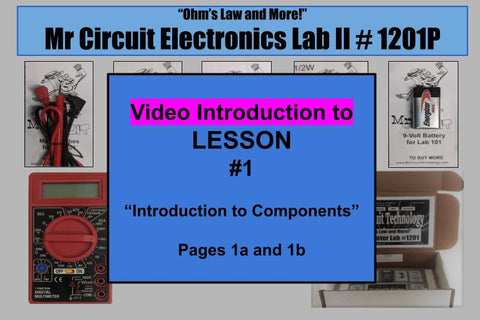THIS WEBSITE IS FOR STEM EDUCATORS and TEACHERS OF TECHNOLOGY. Any questions, call 805-295-1642 - Thank You

# 1201-P Mr Circuit Lab 2 - "Digital Multimeter Fundamentals" LAB - **Meter Included ** Learn Ohm's Law, Watt's Law, and Kirchhoff's Laws. Measure resistance, voltage, and current. Learn the Color-Code for different resistors, and much more.

Regular price
\$39.00
Sale price
\$39.00
Regular price
Sold out
Unit price
per

When you order this Mr Circuit Digital Multimeter Lab,  #1201-P, you will receive a white lab box with all the experiment parts, a digital multimeter, and links to print fully-illustrated lessons.
-----

### LEARN ELECTRONICS AND BECOME A SKILLED TECHNICIAN

Educational Tutorial Lab for:

S.T.E.M. --- Automotive --- HVAC --- Avionics --- Electronics --- Robotics etc.

-------------------------
---------------

Introducing the

Mr Circuit Multimeter Fundamentals Lab 1201-P

#### With this lab, your students will learn electronics technology, and they will have a way of proving that they have. No longer will prospective employers or customers just have to take their word for it.  Upon completion of this lab, you will print out and give them an official signed  8 1/2 x 11" Certificate of Electronics Training that can be added to their resume.  They can display it with pride in their room or office.With this lab, you will work with formulas for Ohm's Law, Watts Law, calculate the equivalent resistance of resistors in series and parallel, use a multimeter to determine the ohms of resistors in series and parallel, calculate resistor wattage, calculate resistor values in LED circuits, calculate and measure current in series and parallel circuits, calculate and measure voltage drops, and more.

You will get practice reading resistor values, current, and voltage on the display of the Digital Multimeter. You will also get more practice using a solderless circuit board to build electronic circuits. (See the whole Skill Set below.).

This lab was created to meet the needs of  S.T.E.M. --- S.T.E.A.M. --- Automotive --- HVAC --- Avionics --- Electronics --- Robotics etc.

I have trained many technical people and one of their biggest needs was to know how to use and interpret the readings on a multimeter.  This knowledge and experience will open up more opportunities at employment and entrepreneurship.

All components necessary for the experiments are included. No extra tools are needed. The lab includes a 9 volt battery power source, a solderless circuit board to build circuits, 10 colored 5mm LEDs (red, green, yellow, blue, clear) and 14 resistors for testing and measuring and, as we said,  a multifunction Digital Multimeter.

This lab can be used for individual instruction as well as a lock-step classroom program.

For students who miss an instruction period, make-up is very easy. The student can watch the video lesson presentation, study the written lesson, and do the experiment.

This lab is also a perfect activity when you have a Substitute Teacher.

There are online video lessons included.Here is the list  of the Lessons in the Mr. Circuit Lab 1201

Lesson #1   REVIEW ELECTRONIC COMPONENTS such as the cell, the battery, the LED, and the resistor

Lesson #2   Connect cells in series and draw a schematic diagram of them

Lesson #3   Work with an LED and its schematic symbol

Lesson #4   REVIEW RESISTOR COLOR CODE and how to use it

Lesson #5   Use the Resistor Color Code with +/- 5% resistors

Lesson #6   Use the Resistor Color Code with +/- 1% resistors

Lesson #7   Take inventory of all the lab parts

Lesson #8   PRACTICE DRAWING A SCHEMATIC diagram of a simple circuit

Lesson #9   Compare schematic symbols to their actual components

Lesson #10 Connect components of a simple circuit

Lesson #11 USE A SOLDERLESS CIRCUIT BOARD  to build circuits

Lesson #12 Build a circuit with a resistor, an LED, and a 9-volt battery.

Lesson #13 Compare brightness of an LED with different resistors in the circuit.

Lesson #14 USE OHM’S LAW with voltage, current, and resistance.

Lesson #15 Calculate the equivalent resistance and current through two resistors in series

Lesson #16 Calculate the equivalent resistance and current through two resistors in parallel

Lesson #17 Compare voltage drops across 5 different colors of LEDs

Lesson #18 Calculate the additional resistance needed with various LEDs

Lesson #19 Light multiple LEDs in parallel and calculate the additional resistances needed

Lesson #20 MEASURE RESISTANCE with a variety of Ohm values on a digital multimeter

Lesson #21 MEASURE CURRENT and set up a digital multimeter to measure different amounts of current

Lesson #22 Build a simple LED circuit and measure the current flowing between resistor and LED

Lesson #23 Measure current in the same circuit between the resistor and the battery

Lesson #24 Measure current in the same circuit between the LED and the resistor

Lesson #25 Build a circuit with a 470 ohm resistor, a red LED, 9-V battery, and measure the current

Lesson #26 Build a circuit with a 1000 ohm resistor, a red LED, 9-V battery, and measure the current

Lesson #27 Build a circuit with a 3300 ohm resistor, a red LED, 9-V battery, and measure the current

Lesson #28 Build a circuit with a 6800 ohm resistor, a red LED, 9-V battery, and measure the current

Lesson #29 MEASURE VOLTS and voltage drops across components with a digital multimeter

Lesson #30 Use Watt’s Law to calculate the wattage rating required of a resistor

Here is the SKILLS SET for the Mr Circuit Lab 1201-P

• Identify the physical appearance and schematic of a single cell, a multicell battery, an LED, and a resistor.

• Explain how cells connected in series form a multicell device called a battery and draw the schematic..

• Describe the schematic symbol for an LED, show the polarity with Anode and Cathode.

• Describe the purpose of the ‘flat side’ and the ‘short lead’ on the LED in reference to the Cathode..

• Understand that the long leg of the LED is the  positive side or the Anode.

• Understand that the beveled edge on an LED is the way to find the Cathode if the LED is in a wired circuit.

• Understand how to use the Resistor Color Code to identify resistor values for both 5% and 1% resistors.

• Take inventory of electronic components and report any discrepancies.

• Draw a simple circuit schematic with symbols and label them including their for polarity.

• Build a basic circuit using the solderless circuit board, the LED, various resistors and a 9-volt battery.

• Match the physical component to their schematic symbol.

• Construct a circuit using a schematic diagram.

• Understand the construction of a solderless circuit board.

• Use Ohm’s Law to calculate voltage, current, and resistance.

• Calculate the equivalent resistance of two resistors in series.

• Calculate the equivalent resistance of two resistors in parallel.

• Identify various voltage drops across different colored LEDs.

• Calculate the additional resistance needed in an LED circuit based on voltage of the power source.

• Connect multiple LEDs in a parallel circuit.

• Measure a variety of values of ohms with a digital multimeter.

• Interpret the reading on the multimeter display in the Ohms function.

• Set up a multimeter to check the current flowing in a circuit.

• Connect the multimeter to a circuit and measure the current.

• Break a series circuit and connect the multimeter to measure the current.

• Explain that the current flowing in a series circuit is the same amount through each component.

• Explain the effect of the amount of current flowing in an LED circuit on the brightness of the LED.

• Set up a multimeter to measure DC voltages.

• Measure DC voltage and voltage drops across components in an active circuit.

• Calculate the minimum wattage needed in a resistor.

We accept Credit / Debit Cards or Public School Purchase Orders.
Thank you from G. Art Gibson, author and educator
Mr Circuit Technology  805-295-1642